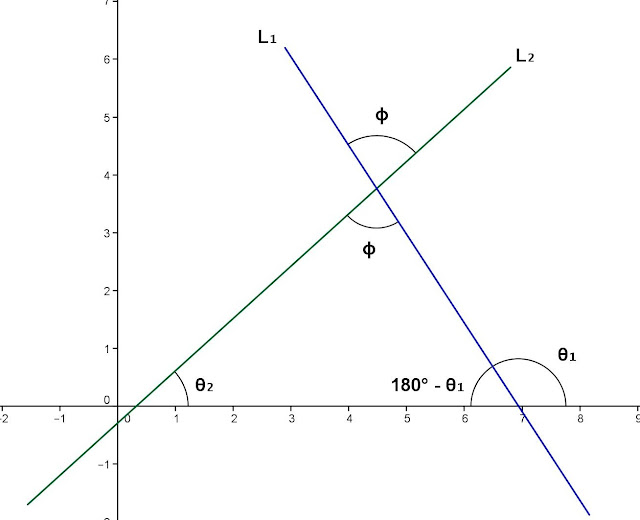## Monday, July 29, 2013

### Derivation - Angle Between Two Lines

Category: Analytic Geometry, Trigonometry

"Published in Newark, California, USA"

Prove that the formula for getting the angle between two lines is

Solution:

To derive the formula for getting the angle between two lines, let's draw the two intersecting lines in Rectangular Coordinate System as follows:Photo by Math Principles in Everyday Life

L1 is the first line that is inclined to the left and θ1 is the angle of inclination of a line in a counterclockwise direction from x-axis.

L2 is the second line that is inclined to the right and θ2 is the angle of inclination of a line in a counterclockwise direction from x-axis.

As you notice in the figure that L1, L2, and x-axis are the sides of a triangle. Let's label further the figure above as followsPhoto by Math Principles in Everyday Life

The sum of the interior angles of a triangle is calculated as follows

Take tangent on both sides of the equation, we have

Substitute the right side of the equation with the slope of two lines as followsPhoto by Math Principles in Everyday Life

The slope of L1 is

The slope of L2 is

Therefore, the formula for getting the angle between two lines is